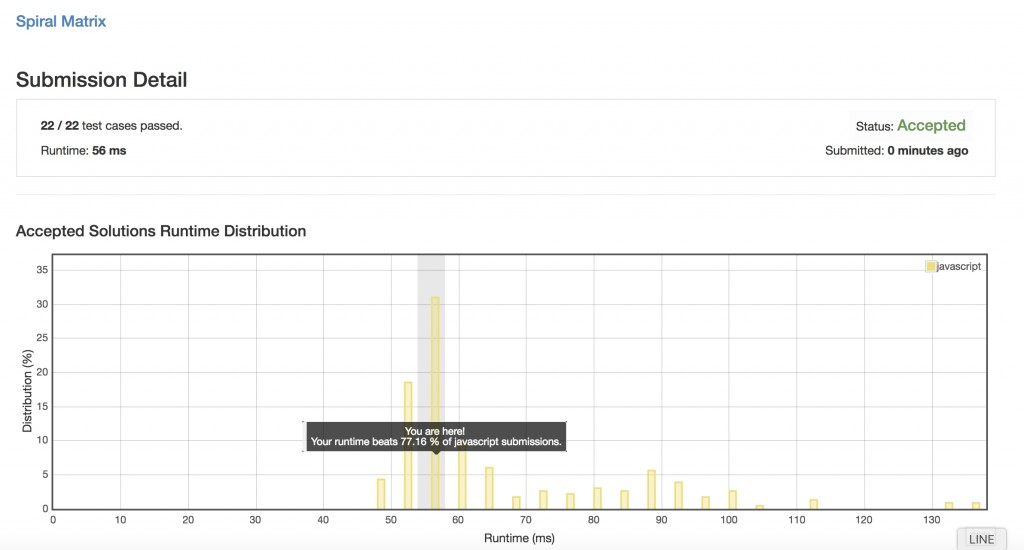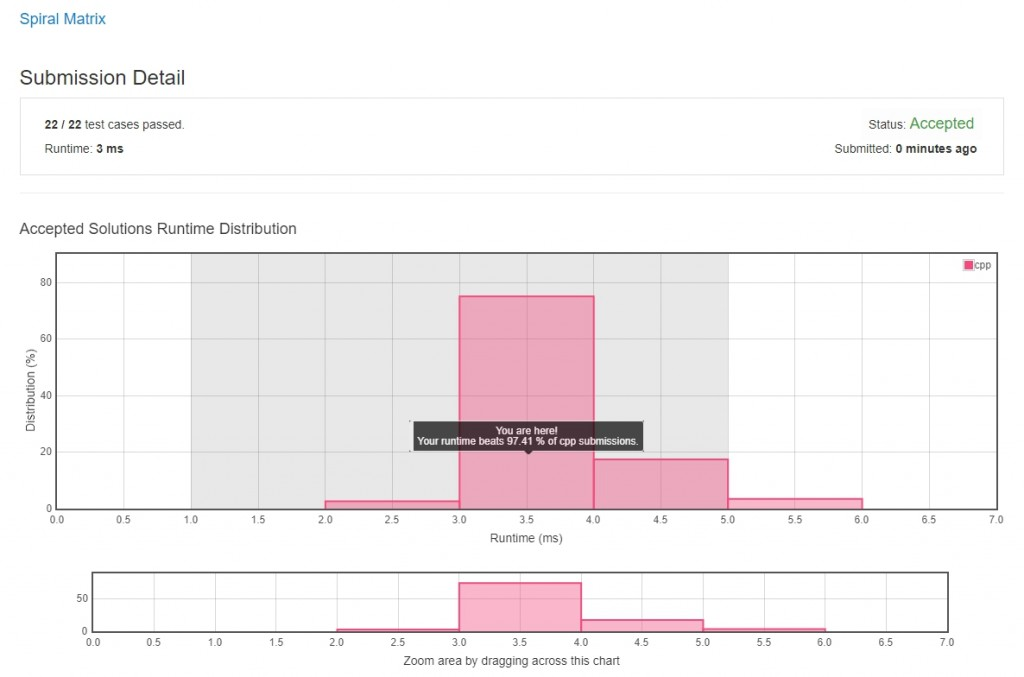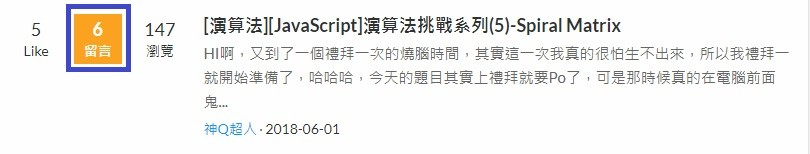#7

## [演算法][JavaScript]演算法挑戰系列(5)-Spiral Matrix

HI啊，又到了一個禮拜一次的燒腦時間，其實這一次我真的很怕生不出來，所以我禮拜一就開始準備了，哈哈哈，今天的題目其實上禮拜就要Po了，可是那時候真的在電腦前面鬼打牆很久，就直接換到Hard難度的SQL題目了，所以這篇算是來還債的XD，感謝大家持續支持這個系列1.傳入以下陣列：
[
[1,2,3],
[4,5,6],
[7,8,9]
]

2.傳入以下陣列：
[
[1, 2, 3 ],
[4, 5, 6 ],
[7, 8, 9 ],
[10,11,12]
]

``````var spiralOrder = function(matrix) {

//如果傳進來是空陣列就回傳空陣列
if(matrix.length === 0){
return [];
}

//用來裝解答
let ans = [];
//陣列中所有的數字有幾個
let indexCount = matrix.length * matrix.length;
//用來記錄目前的流程(0:上,1:右,2:下,3:左)
let seq = 0;
//指定回傳的位置值
//第一維度是x，第二維度是y(等等進入迴圈後y會先+1所以初始值為-1)
let x = 0;
let y = -1;
//跑迴圈(如果解答陣列中的長度小於matrix的所有元素就繼續跑，因為ans最後的陣列長度一定會和matrix一樣)
while(ans.length < indexCount){
//指定位置
changeIndex(1);
//宣告一個變數用來放目前要抓的值
let nowValue;
//取值用try迴圈包起來
try{
//用目前指定的位置把值從陣列取出來
nowValue = matrix[x][y];
}
catch(err){
//如果超出該陣列範圍就回傳undefined
nowValue = undefined;
};

//如果回傳的值不在ans裡面又不是undefined就把他放進ans中
if(ans.indexOf(nowValue) ===- 1 && nowValue !== undefined){
ans.push(nowValue);
}
else{
/*這裡是因為如果取出來undefuned或是重複的值，
要先把這一次迴圈加的位置減回來，
等於回復進入這一次迴圈前的狀態。*/
changeIndex(-1);
//這裡用來變動流程，依序由上:0,右:1,下:2,左:3去取值
if(seq === 3){
seq = 0;
}
else{
seq+=1;
}
};
};

//用來變動位置，index傳入變動值
function changeIndex (index){
//確認目前流程，依照流程來做變動(如果傳進-1就是回復之前的狀態)
switch(seq){
case 0:{
//如果是0(上)，就是往右一格，所以y+1
y+=index;
break;
};
case 1:{
//如果是1(右)，就是往下一格，所以x+1
x+=index;
break;
};
case 2:{
//如果是2(下)，就是往左一格，所以y-1
y-=index;
break;
};
case 3:{
//如果是3(左)，就是往上一格，所以x-1
x-=index;
break;
};
};
};

//最後回傳裝完所有值的陣列
return ans;

};
````````````var spiralOrder = function (matrix) {

"use strict";

//宣告一個新陣列
var res = [];

//一個function來判斷陣列內是否還有值，如果有就回傳false，沒有就true
var isEmpty = function isEmpty() {
return matrix.length === 0 || matrix.length === 0;
};

//如果他還不是空的就繼續跑迴圈
while (!isEmpty()) {

//抓出matrix的第一個陣列位置，並把他放進res中(處理上面的部分)
res = res.concat(matrix.shift());

//確認目標陣列是否為空，如果是空的就跳出
if (isEmpty()) break;

//去跑迴圈，經過目前的所有位置
for (var i = 0; i < matrix.length; i++) {
//把每個位置的最後一個值抓出來放進res中(處理右邊的部分)
res.push(matrix[i].pop());
}

//確認目標陣列是否為空，如果是空的就跳出
if (isEmpty()) break;

//把最後一個位置抓出來，反轉後放進res中(處理下面的部份)
res = res.concat(matrix.pop().reverse());

//確認目標陣列是否為空，如果是空的就跳出
if (isEmpty()) break;

//去跑迴圈，經過目前的所有位置
for (var _i = matrix.length - 1; _i >= 0; _i--) {
//把每個位置的第一個值抓出來放進res中(處理左邊的部分)
res.push(matrix[_i].shift());
}
}

//重複以上流程，直到目標陣列被處理到空了為止。
return res;
};
``````

### 2 則留言

2``````class Solution
{
public:
vector<int> spiralOrder(vector<vector<int>> &matrix)
{
//分別對應，左->下->右->上
int dir_x = {0, 1, 0, -1};
int dir_y = {1, 0, -1, 0};

//取得矩陣邊長
int size_x = matrix.size();
int size_y = size_x == 0 ? 0 : matrix.size();

vector<int> output(size_x * size_y);

int current = 0;   //紀錄回傳結果的索引
int index = 0;     //紀錄行走方向的索引
int x = 0;         //當前的 x 座標
int y = 0;         //當前的 y 座標

for (int i = 0; i < size_x * size_y; i++)
{
//將當前座標的值寫入結果，並在寫入後設為 0
output[current++] = matrix[x][y];
matrix[x][y] = 0;

//前進
x += dir_x[index];
y += dir_y[index];

//遇到邊界或已走過的座標
if (x < 0 || x >= size_x ||
y < 0 || y >= size_y ||
matrix[x][y] == 0)
{
//退回
x -= dir_x[index];
y -= dir_y[index];

//轉彎
index = (index + 1) % 4;

//前進
x += dir_x[index];
y += dir_y[index];
}
}
return output;
}
};
``````3

``````static int[] GetArray(int[][] array)
{
int length1 = array.Length;
int length2 = array.Length;

//先檢查是不是全部都一樣大小
for (int i = 0; i < length1; i++)
if (array[i].Length != length2)
return null;

int[] ans = new int[length1 * length2];

EDirection dir = EDirection.RIGHT;
int x = 0;
int y = 0;
int index = 0;

while(index < length1 * length2)
{
ans[index] = array[y][x];
switch(dir)
{
case EDirection.RIGHT: //向右
if (x + y == length2 - 1)
{
dir = EDirection.DOWN;
y += 1;
}
else
x += 1;
break;
case EDirection.DOWN: //向下
if (x - y == length2 - length1)
{
dir = EDirection.LEFT;
x -= 1;
}
else
y += 1;
break;
case EDirection.LEFT: //向左
if (x + y == length1 - 1)
{
dir = EDirection.UP;
y -= 1;
}
else
x -= 1;
break;
case EDirection.UP: //向上
if (y == x + 1)
{
dir = EDirection.RIGHT;
x += 1;
}
else
y -= 1;
break;
}
index++;
}

return ans;
}
``````

``````for (int i = 0; i < length1; i++)
if (array[i].Length != length2)
return null;
``````

``````EDirection dir = EDirection.RIGHT;
``````[
[1, 2, 3 ],
[4, 5, 6 ],
[7, 8, 9 ],
[10,11,12]
]ㄛ對啊！因為一場意外